# Quantum cryptography by Girisha Shankar, Sr. Manager, Cisco

13 de Apr de 2019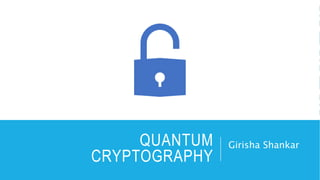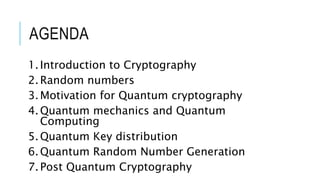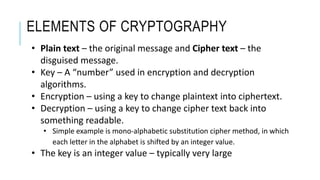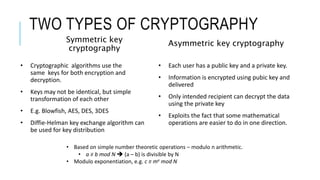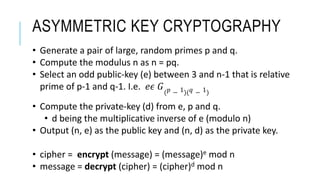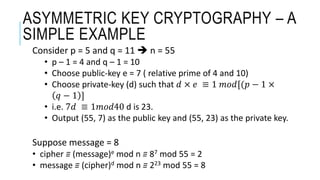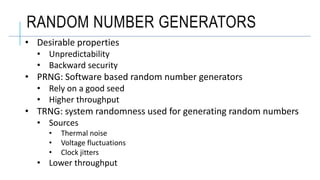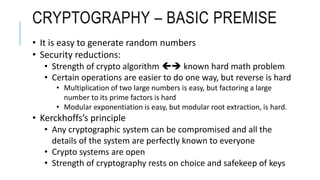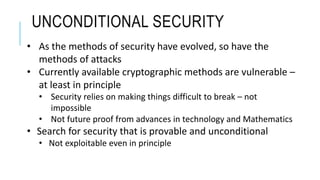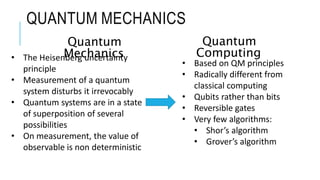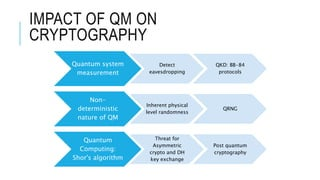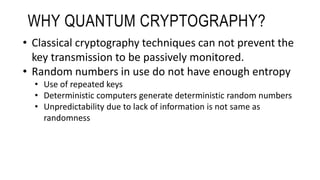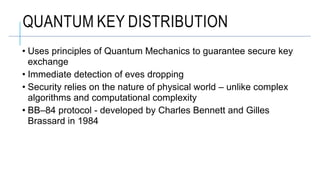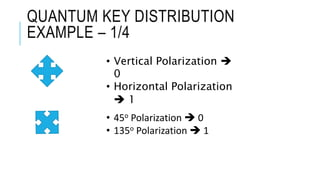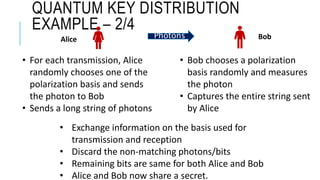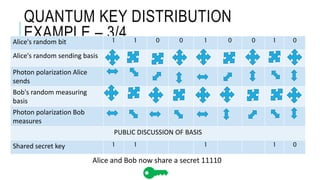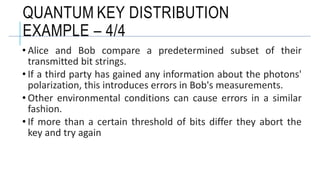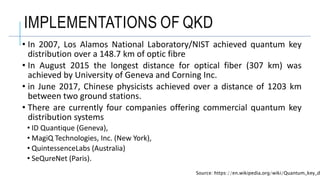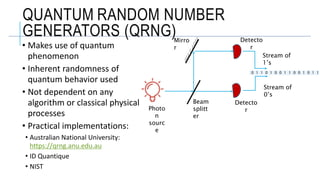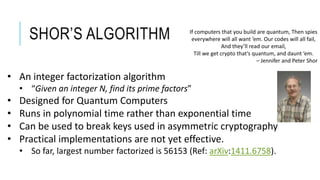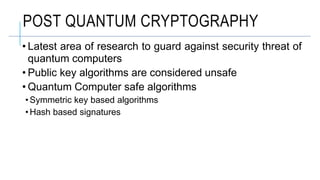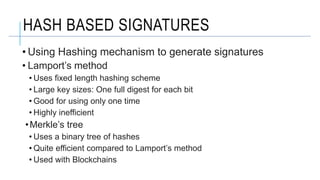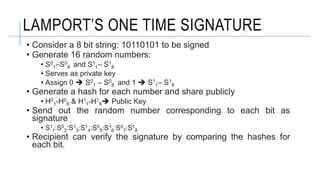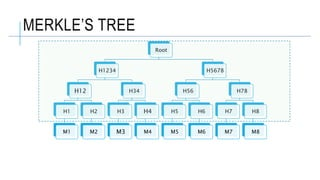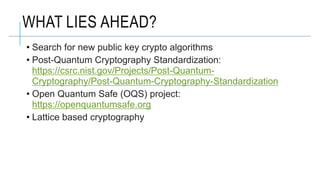1 de 25

### Quantum cryptography by Girisha Shankar, Sr. Manager, Cisco

1. QUANTUM CRYPTOGRAPHY Girisha Shankar
2. AGENDA 1.Introduction to Cryptography 2.Random numbers 3.Motivation for Quantum cryptography 4.Quantum mechanics and Quantum Computing 5.Quantum Key distribution 6.Quantum Random Number Generation 7.Post Quantum Cryptography
3. ELEMENTS OF CRYPTOGRAPHY • Plain text – the original message and Cipher text – the disguised message. • Key – A “number” used in encryption and decryption algorithms. • Encryption – using a key to change plaintext into ciphertext. • Decryption – using a key to change cipher text back into something readable. • Simple example is mono-alphabetic substitution cipher method, in which each letter in the alphabet is shifted by an integer value. • The key is an integer value – typically very large
4. TWO TYPES OF CRYPTOGRAPHY Symmetric key cryptography • Cryptographic algorithms use the same keys for both encryption and decryption. • Keys may not be identical, but simple transformation of each other • E.g. Blowfish, AES, DES, 3DES • Diffie-Helman key exchange algorithm can be used for key distribution Asymmetric key cryptography • Each user has a public key and a private key. • Information is encrypted using pubic key and delivered • Only intended recipient can decrypt the data using the private key • Exploits the fact that some mathematical operations are easier to do in one direction. • Based on simple number theoretic operations – modulo n arithmetic. • a ≡ b mod N  (a – b) is divisible by N • Modulo exponentiation, e.g. c ≡ me mod N
5. ASYMMETRIC KEY CRYPTOGRAPHY • Generate a pair of large, random primes p and q. • Compute the modulus n as n = pq. • Select an odd public-key (e) between 3 and n-1 that is relative prime of p-1 and q-1. I.e. 𝑒𝜖 𝐺( 𝑝 − 1)( 𝑞 − 1) • Compute the private-key (d) from e, p and q. • d being the multiplicative inverse of e (modulo n) • Output (n, e) as the public key and (n, d) as the private key. • cipher = encrypt (message) = (message)e mod n • message = decrypt (cipher) = (cipher)d mod n
6. ASYMMETRIC KEY CRYPTOGRAPHY – A SIMPLE EXAMPLE Consider p = 5 and q = 11  n = 55 • p – 1 = 4 and q – 1 = 10 • Choose public-key e = 7 ( relative prime of 4 and 10) • Choose private-key (d) such that 𝑑 × 𝑒 ≡ 1 𝑚𝑜𝑑[(𝑝 − 1 × 𝑞 − 1 ] • i.e. 7𝑑 ≡ 1𝑚𝑜𝑑40 d is 23. • Output (55, 7) as the public key and (55, 23) as the private key. Suppose message = 8 • cipher ≡ (message)e mod n ≡ 87 mod 55 = 2 • message ≡ (cipher)d mod n ≡ 223 mod 55 = 8
7. RANDOM NUMBER GENERATORS • Desirable properties • Unpredictability • Backward security • PRNG: Software based random number generators • Rely on a good seed • Higher throughput • TRNG: system randomness used for generating random numbers • Sources • Thermal noise • Voltage fluctuations • Clock jitters • Lower throughput
8. CRYPTOGRAPHY – BASIC PREMISE • It is easy to generate random numbers • Security reductions: • Strength of crypto algorithm  known hard math problem • Certain operations are easier to do one way, but reverse is hard • Multiplication of two large numbers is easy, but factoring a large number to its prime factors is hard • Modular exponentiation is easy, but modular root extraction, is hard. • Kerckhoffs’s principle • Any cryptographic system can be compromised and all the details of the system are perfectly known to everyone • Crypto systems are open • Strength of cryptography rests on choice and safekeep of keys
9. UNCONDITIONAL SECURITY • As the methods of security have evolved, so have the methods of attacks • Currently available cryptographic methods are vulnerable – at least in principle • Security relies on making things difficult to break – not impossible • Not future proof from advances in technology and Mathematics • Search for security that is provable and unconditional • Not exploitable even in principle
10. QUANTUM MECHANICS • The Heisenberg uncertainty principle • Measurement of a quantum system disturbs it irrevocably • Quantum systems are in a state of superposition of several possibilities • On measurement, the value of observable is non deterministic • Based on QM principles • Radically different from classical computing • Qubits rather than bits • Reversible gates • Very few algorithms: • Shor’s algorithm • Grover’s algorithm Quantum Mechanics Quantum Computing
11. IMPACT OF QM ON CRYPTOGRAPHY Quantum system measurement Detect eavesdropping QKD: BB-84 protocols Non- deterministic nature of QM Inherent physical level randomness QRNG Quantum Computing: Shor's algorithm Threat for Asymmetric crypto and DH key exchange Post quantum cryptography
12. • Classical cryptography techniques can not prevent the key transmission to be passively monitored. • Random numbers in use do not have enough entropy • Use of repeated keys • Deterministic computers generate deterministic random numbers • Unpredictability due to lack of information is not same as randomness WHY QUANTUM CRYPTOGRAPHY?
13. • Uses principles of Quantum Mechanics to guarantee secure key exchange • Immediate detection of eves dropping • Security relies on the nature of physical world – unlike complex algorithms and computational complexity • BB–84 protocol - developed by Charles Bennett and Gilles Brassard in 1984 QUANTUM KEY DISTRIBUTION
14. QUANTUM KEY DISTRIBUTION EXAMPLE – 1/4 • Vertical Polarization  0 • Horizontal Polarization  1 • 45o Polarization  0 • 135o Polarization  1
15. QUANTUM KEY DISTRIBUTION EXAMPLE – 2/4 • For each transmission, Alice randomly chooses one of the polarization basis and sends the photon to Bob • Sends a long string of photons • Bob chooses a polarization basis randomly and measures the photon • Captures the entire string sent by Alice Alice BobPhotons • Exchange information on the basis used for transmission and reception • Discard the non-matching photons/bits • Remaining bits are same for both Alice and Bob • Alice and Bob now share a secret.
16. QUANTUM KEY DISTRIBUTION EXAMPLE – 3/4Alice's random bit 1 1 0 0 1 0 0 1 0 Alice's random sending basis Photon polarization Alice sends Bob's random measuring basis Photon polarization Bob measures PUBLIC DISCUSSION OF BASIS Shared secret key 1 1 1 1 0 Alice and Bob now share a secret 11110
17. • Alice and Bob compare a predetermined subset of their transmitted bit strings. • If a third party has gained any information about the photons' polarization, this introduces errors in Bob's measurements. • Other environmental conditions can cause errors in a similar fashion. • If more than a certain threshold of bits differ they abort the key and try again QUANTUM KEY DISTRIBUTION EXAMPLE – 4/4
18. IMPLEMENTATIONS OF QKD • In 2007, Los Alamos National Laboratory/NIST achieved quantum key distribution over a 148.7 km of optic fibre • In August 2015 the longest distance for optical fiber (307 km) was achieved by University of Geneva and Corning Inc. • in June 2017, Chinese physicists achieved over a distance of 1203 km between two ground stations. • There are currently four companies offering commercial quantum key distribution systems • ID Quantique (Geneva), • MagiQ Technologies, Inc. (New York), • QuintessenceLabs (Australia) • SeQureNet (Paris). Source: https://en.wikipedia.org/wiki/Quantum_key_d
19. Stream of 1’s Stream of 0’s Mirro r Beam splitt er Photo n sourc e Detecto r Detecto r QUANTUM RANDOM NUMBER GENERATORS (QRNG) • Makes use of quantum phenomenon • Inherent randomness of quantum behavior used • Not dependent on any algorithm or classical physical processes • Practical implementations: • Australian National University: https://qrng.anu.edu.au • ID Quantique • NIST 0 1 1 0 1 0 0 1 1 0 0 1 0 1 1
20. SHOR’S ALGORITHM If computers that you build are quantum, Then spies everywhere will all want ’em. Our codes will all fail, And they’ll read our email, Till we get crypto that’s quantum, and daunt ’em. – Jennifer and Peter Shor • An integer factorization algorithm • “Given an integer N, find its prime factors” • Designed for Quantum Computers • Runs in polynomial time rather than exponential time • Can be used to break keys used in asymmetric cryptography • Practical implementations are not yet effective. • So far, largest number factorized is 56153 (Ref: arXiv:1411.6758).
21. POST QUANTUM CRYPTOGRAPHY • Latest area of research to guard against security threat of quantum computers • Public key algorithms are considered unsafe • Quantum Computer safe algorithms • Symmetric key based algorithms • Hash based signatures
22. HASH BASED SIGNATURES • Using Hashing mechanism to generate signatures • Lamport’s method • Uses fixed length hashing scheme • Large key sizes: One full digest for each bit • Good for using only one time • Highly inefficient •Merkle’s tree • Uses a binary tree of hashes • Quite efficient compared to Lamport’s method • Used with Blockchains
23. LAMPORT’S ONE TIME SIGNATURE • Consider a 8 bit string: 10110101 to be signed • Generate 16 random numbers: • S0 1–S0 8 and S1 1– S1 8 • Serves as private key • Assign 0  S0 1 – S0 8 and 1  S1 1– S1 8 • Generate a hash for each number and share publicly • H0 1-H0 8 & H1 1-H1 8 Public Key • Send out the random number corresponding to each bit as signature • S1 1:S0 2:S1 3:S1 4:S0 5:S1 6:S0 7:S1 8 • Recipient can verify the signature by comparing the hashes for each bit.
24. MERKLE’S TREE Root H1234 H12 H1 M1 H2 M2 H34 H3 M3 H4 M4 H5678 H56 H5 M5 H6 M6 H78 H7 M7 H8 M8
25. WHAT LIES AHEAD? • Search for new public key crypto algorithms • Post-Quantum Cryptography Standardization: https://csrc.nist.gov/Projects/Post-Quantum- Cryptography/Post-Quantum-Cryptography-Standardization • Open Quantum Safe (OQS) project: https://openquantumsafe.org • Lattice based cryptography